# Units in MathML

## W3C Working Group Note 10 November 2003

This version:
http://www.w3.org/TR/2003/NOTE-mathml-units-20031110/
http://www.w3.org/TR/mathml-units/
Previous version:
This is the first version of this Note.
Editors:
Douglas Wilhelm Harder - Invited Expert, University of Waterloo
Stan Devitt - Invited Expert, StratumTek

## Abstract

MathML is an XML application for describing mathematical notation, capturing both its structure and content. As such, its scope does not extend to include units - determinate quantities adopted as standards of measure - which nevertheless, by their very nature, occur in an applied mathematical setting. This Note makes recommendations and suggestions for how units can be incorporated into MathML.

## Status of this Document

This section describes the status of this document at the time of its publication. Other documents may supersede this document. A list of current W3C publications and the latest revision of this technical report can be found in the W3C technical reports index at http://www.w3.org/TR/.

This document makes recommendations and suggestions for how to work with units in MathML. Please report comments and errors in this document to www-math@w3.org.

This document has been produced by the W3C Math Working Group as part of the W3C Math Activity (Activity statement). The goals of the Working Group are discussed in the Working Group Charter. A list of participants in the W3C Math Working Group is available.

Publication as a Working Group Note does not imply endorsement by the W3C Membership. This is a draft document and may be updated, replaced or obsoleted by other documents at any time. It is inappropriate to cite this document as other than work in progress. Patent disclosures relevant to this Note may be found on the Math Working Group's patent disclosure page.

## 1 Introduction

The support of units within MathML [MathML2] is of primary interest to those wishing to use MathML to encode measured data. This Note presents a recommendation for how to include units in any MathML expression. It deals with both the presentation and content representations of units.

The primary reference for this document is the IEEE/ASTM SI 10-1997 Standard for the Use of the International System of Units (SI): The Modern Metric System[ieee-astm], hereafter referred to as the standard. This Note only interprets what is defined in the standard and should not be used as a replacement.

### 1.1 Goals

The goals of this Note are to provide suggestions and recommendations for encoding the presentation, identification, and inclusion of units in both Presentation and Content MathML.

### 1.2 Definitions

The term unit name refers to the actual name of a unit (for example, meter, feet, torr), while unit symbol refers to usually a smaller number of characters used to represent the associated unit (for example, m, ft, Torr). Unit symbols are not abbreviations, and abbreviations should never be used as symbols. For example, use A and not amp. For archaic units with no defined symbol, use the unit name as the unit symbol.

A compound unit is a unit which is written as a product of powers of unit symbols and constants.

## 2 Display of Units in Presentation MathML

Section 3.5 of the standard is devoted to the style and usage of writing unit names and symbols. The following highlights the most relevant points brought up in this section and suggests possible interpretations in MathML.

When writing compound unit names, the standard recommends that they be written in full English with standard pluralization and spacing, for example, kilograms per cubic meter and meters per second squared. MathML is concerned with the incorporation of units into mathematical expressions, and so the unit names are often inappropriate. It is recommended instead that the unit symbols are used at all times.

Unit symbols are written in roman (upright) type, are not altered in the plural, are not followed by a period except at the end of a sentence, and no space is left between a prefix and a unit symbol. This is accomplished in MathML by using the `mi` element. Single character symbols must be qualified by setting the `mathvariant` attribute to `normal` as otherwise they would be italicized. For example,

`<mi mathvariant='normal'>m</mi> `

The standard recommends that a raised dot is used to indicate the product of two units. In Presentation MathML, this may be done through the use of the mathematical operator `<mo>&middot;</mo>`. For example, the newton meter may be expressed as

```<mrow>
<mi mathvariant='normal' class='MathML-Unit'>N</mi>
<mo>&middot;</mo>
<mi mathvariant='normal' class='MathML-Unit'>m</mi>
</mrow> ```

A quotient of two or more units may be expressed in one of the following ways:

MathML SourceDisplayDescription
`<mi mathvariant='normal' class='MathML-Unit'>J</mi> <mo>/</mo> <mi mathvariant='normal' class='MathML-Unit'>kg</mi>`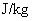J/kg
`<mi mathvariant='normal' class='MathML-Unit'>J</mi> <mo>&middot;</mo> <msup><mi mathvariant='normal' class='MathML-Unit'>kg</mi> <mn>-1</mn></msup><mfrac>`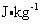J·kg-1
`<mfrac><mi mathvariant='normal' class='MathML-Unit'>J</mi> <mi mathvariant='normal' class='MathML-Unit'>kg</mi></mfrac>`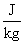the ratio of J over kg

In representing a quotient, do not use more than one solidus (/) in the same expression unless parentheses are inserted to avoid ambiguity.

In expressing a quantity containing a value and a unit symbol, a space shall be left between them. In MathML this is achieved by using the mathematical operator `<mo rspace="thickmathspace">&InvisibleTimes;</mo>`. If the default spacing used for `&InvisibleTimes;` is not acceptable (it is often zero), extra space is added in MathML with the `rspace` attribute. For example, `35 mm` may be expressed as

```<mrow>
<mn>35</mn> <mo rspace='thickmathspace'>&InvisibleTimes;</mo>
<mi mathvariant='normal' class='MathML-Unit'>mm</mi>
</mrow> ```

In expressing a quantity containing a value and a compound unit, the unit should remain distinct from the value. For example, in expressing 10 kg·m/s, one would use:

```<mrow>
<mn>10</mn> <mo rspace='thickmathspace'>&InvisibleTimes;</mo>
<mfrac>
<mrow>
<mi mathvariant='normal' class='MathML-Unit'>kg</mi> <mo>&middot;</mo> <mi
mathvariant='normal' class='MathML-Unit'>m</mi>
</mrow> <mi mathvariant='normal' class='MathML-Unit'>s</mi>
</mfrac>
</mrow>```

and not

```<mfrac>
<mrow>
<mn>10</mn> <mo rspace='thickmathspace'>&InvisibleTimes;</mo>
<mi>kg</mi> <mo>&middot;</mo> <mi mathvariant='normal'>m</mi>
</mrow> <mi mathvariant='normal'>s</mi>
</mfrac> ```

For both unit symbols and compound units, the unit should be the last term in the product.

### 2.1 Exceptions

As MathML may be used in the display of intermediate steps of a calculation, not all of the above rules may apply. For example, in finding the speed of a body which moved thirty meters in five seconds, the Presentation MathML markup may be:

```<mrow>
<mfrac>
<mrow>
<mn>30</mn>
<mo rspace='thickmathspace'>&InvisibleTimes;</mo>
<mi mathvariant='normal' class='MathML-Unit'>m</mi>
</mrow>
<mrow>
<mn>5</mn>
<mo rspace='thickmathspace'>&InvisibleTimes;</mo>
<mi mathvariant='normal' class='MathML-Unit'>s</mi>
</mrow>
</mfrac>
<mo>=</mo>
<mn>6</mn>
<mo rspace='thickmathspace'>&InvisibleTimes;</mo>
<mfrac>
<mi mathvariant='normal' class='MathML-Unit'>m</mi>
<mi mathvariant='normal' class='MathML-Unit'>s</mi>
</mfrac>
</mrow> ```

which displays as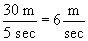Certain non-SI units have, through common usage, symbols which differ in the plural. For example, the pound may be represented by the symbol `lb` or `lbs` , depending on the amount being measured. Similarly, 3.2 yards is often written 3.2 yds.

The symbol kWh for the kilowatthour instead of kW·h is also acceptable.

Exceptions to the requirement that a space separate the quantity and unit are the symbols for degree, minute, and second of plane angle. In this case, it would be appropriate to use the `mo` operator. For example,

```<mn>32</mn>
<mo>&InvisibleTimes;</mo>
<mi mathvariant='normal' class='MathML-Unit'>°</mi>
<mn>18</mn>
<mo>&InvisibleTimes;</mo>
<mi mathvariant='normal' class='MathML-Unit'>'</mi>
<mn>54</mn>
<mo>&InvisibleTimes;</mo>
<mi mathvariant='normal' class='MathML-Unit'>"</mi>```

## 3 Inclusion of Units in Content MathML

In expressing a quantity with units, as with Presentation MathML, it is recommended that the unit be the last child of an `apply` element which has a `times` element as the first child. It is also preferable if compound units are kept separate as a nested apply at the end of a product.

## 4 Identifying Units in Presentation MathML

The presentation element `mi` does not take any attributes which may be used to indicate that it represents a unit. Content MathML must be used to identify individual or compound units.

## 5 Identifying Unit Symbols in Content MathML

MathML 2.0 introduced externally defined symbols with the `csymbol` element. This element allows the author to use identifiers with externally defined semantics.

The attribute `definitionURL` is a pointer to the external definition of the semantics of the symbol. The attribute `encoding` gives the syntax of the definition pointed to by the definition URL. Thus, the `csymbol` content element as such is the appropriate tool for identifying a symbol as being a unit.

The `csymbol` element should not be used to encapsulate a compound unit. For example, the following unit of speed should not be constructed using a single `csymbol` element: `<csymbol>centimeters per second</csymbol>` or `<csymbol>cm/s</csymbol>`.

Such compound units should be represented by explicit products or quotients of simple units.

### 5.1 Element Content

The content of the `csymbol` element may consist of any encoding which is desired to represent the unit and is not restricted in any way beyond those already set in the MathML 2.0 specification.

The symbol g0 or gn is often used to represent the unit of acceleration due to freefall. The semantics are already defined by the attributes, and thus the content may be used to indicate the display of this unit. For example,

`<csymbol definitionURL='http://.../units/gravity'><msub><mi>g</mi><mi>n</mi></msub></csymbol>`

### 5.2 Encoding Attribute

The `encoding` attribute is optional and is used to help parse the actual defintion. It is only needed if the intention is to actually interpret the definitions by machine.

### 5.3 Definition URL Attribute

The `definitionURL` attribute may be used to uniquely identify a unit. The following is a recommendation for a unique definition URL.

The definition URL is made up of two to five parts:

`http://base/units/unit name[/context][/country][#prefix]`

where the context, country, and prefix are optional. The use of these parts is described below:

#### 5.3.1 Base

To this point, no fixed URL has been chosen to represent the base of these definition URLs, so for the remainder of this Note, the base will be indicated by ellipsis: `...`. For example,

`<csymbol definitionURL='http://.../units/meter'>m</csymbol>`

#### 5.3.2 Unit Name

The unit name is spelled in full without any prefix. With the myriad of spellings available for each unit name, even within SI, it is recommended that the American English spelling with standard translations to lower case ASCII characters be used wherever possible. For example, the German Fuß and the Ångström should use the definition URLs `http://.../units/foot/de` and `http://.../units/angstrom`, respectively.

In cases where the (nonstandard) unit symbol is an abbreviation of a compound unit name, the unit symbol should be used in place of the unit name. For example, gallons per minute and revolutions per minute should use the definition URLs should use the definition URLs `http://.../units/gpm` and `http://.../units/rpm` , respectively.

The unit name was chosen over the unit symbol for the definition URL as the name is less likely to conflict with other unit names, and unit names tend to be more easily converted to ASCII. For example, the symbol for the ohm is Ω (&Omega;).

#### 5.3.3 Context

The context is used only where the name is insufficient to properly qualify the unit either through common usage or an international or scientific agreement. No SI base units, derived units with special names and symbols, or derived units with special names admitted for reasons of safeguarding human health requires a context as they are understood to be the default. Of the units in use with and in temporary use with SI, the only which require a context are the angular minute and second, `http://.../units/minute/angular` and `http://.../units/second/angular`, respectively.

An example of where a context is necessary is the U.S. survey mile. The default international mile has the definition URL `http://.../units/mile` whereas the U.S. survey mile would use the definition URL `http://.../units/mile/survey/us`. In this case, the context is necessary as the standard U.S. mile is equal to the international mile.

Over the years, the definitions of the various SI units have been modified. In most cases, the definitions have been made more exact, so previous measurements are still valid up to the precision of the former definition. The one exception to this is the definition of the liter. In 1901, the liter was defined as the unit of volume occupied by a mass of one kilogram of pure water at its maximum density and at standard atmospheric pressure. The definition of the liter was changed in 1964 to be a special name for the cubic decimeter. These two definitions differ by approximately 28 parts per million, and thus it is necessary to differentiate between these two units. The context of the original liter could be the year the definition was made, so the definition URL would be `http://.../units/liter/1901`.

A date may be used in other cases where a definition has changed, where the date refers to the year the definition in question was made.

In some cases, a unit may have multiple definitions which may be differentiated by the dimension. In this case, the dimension could be used as the context. For example, the langley may either be defined as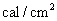(surface energy density) or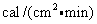(surface power density). The definition URLs for these two units may be `http://.../units/langley/surface_energy_density` and `http://.../units/langley/surface_power_density`, respectively.

#### 5.3.4 Country Code

By default, the country is referred to by the ISO 3166 country codes. In the case of historical units, where possible, the modern successor of that country should be used. Where this is not possible, ISO 3166-2 Regional codes may be used, again, if possible.

For example, the definition URL of the Scottish mile would be `http://.../units/mile/gb-sct`.

This breaks down when referring to ancient units. It would be nonsensical to refer to the Roman mile as an Italian mile. In these cases, the adjective form of the civilization is used. Some examples are given below:

• arabic

• athenian

• egyptian

• greek

• hebrew

• ionian

• persian

• prussian

• rabbinical

• roman

• talmudic

#### 5.3.5 Prefix

SI accomodates decimal multiples and submultiples of units to be represented by placing prefixes in front of the symbols. The IEC introduced prefixes to represent binary multiples of units. A unit with a prefix is represented by appending the prefix to the definition URL using the fragment identifier `#`. For the prefix `μ` (micro) use the recommended ASCII equivalent, `u`.

Examples:

Unit NameSymbolRecommended Definition URL
centimetercm`http://.../units/meter#c`
micrometerμm`http://.../units/meter#u`
kilogramkg`http://.../units/gram#k`
kibibyte (1024 bytes)KiB`http://.../units/byte#Ki`

A full list of prefixes are given in B.2 IEC Prefixes. Unlike other parts of the definition URL, the prefixes should be capitalized where necessary.

## 6 Facilitating the Conversion of Units in MathML

A unit is a standard of measurement. In order to convert, for example, 1.35 dynes to newtons, two pieces of information are necessary:

1. The dimension of the unit, and

2. Either:

1. The associated system of units, or

2. The conversion to a known system of units.

At this point, the measurement can be converted to any other unit of equal dimension.

Suppose we wish to convert 15.3 kilometers per hour to feet per second. Both are measurements of speed, the second being the unit of speed in the foot-pound-second system of units. If we know that the conversion of km/h to SI is approximately 0.277778 m/s, then the conversion from m/s is the conversion of m to ft over the conversion of s to s, that is, 3.28084. Thus, the conversion is 15.3×0.277778×3.28084 ft/s = 13.9436 ft/s.

Suppose we want to convert 3.532 calorie hours to erg seconds. This problem may be reduced to converting the first unit to a common system of units, say SI, and then converting the corresponding SI unit to cgs system of units.

Given that cal·h and erg·s are units of action (the dimension), if we know that the conversion of 1 cal·s to SI is 15062.4 kg·m2/s. The conversion from SI to cgs is a multiplication by 107. Thus 3.532 cal·h = 3.532×15062.4×107erg·s = 5.32e11 erg·s.

Again, consider converting 83.7 statvolts per centimeter to volts per meter. This problem may be reduced to converting a unit in the electrostatic system of units (emu) to SI.

Given that sV/cm is a unit of electric field strength, we may take the product of the conversions of corresponding units of mass, length time, and electric current to get the conversion to SI. This product is 10-3×10-2/(13×2997924580-1) = 29979.2458, and therefore the conversion to SI of 87.3 statV/cm is 29979.2458 V/m.

It is always possible to determine all of this information by interpreting the MathML appropriately, although by transmitting plain MathML the task may be quite difficult. Even a unit as simple as cm/s may be stored in any of three ways:

```<apply>
<divide/>
<csymbol definitionURL='http://.../units/meter#c>cm</csymbol>
<csymbol definitionURL='http://.../units/second>s</csymbol>
</apply>

<apply>
<times/>
<csymbol definitionURL='http://.../units/meter#c>cm</csymbol>
<apply>
<power/>
<csymbol definitionURL='http://.../units/second>s</csymbol>
<cn type='integer'>-1</cn>
</apply>
</apply>

<csymbol definitionURL='http://.../units/kyne'>kyn</csymbol>```

In general, it is quite difficult to pick a unit from an expression. This section describes a method for facilitating the transmission of unit information by making use of the `semantics` element.

Each `semantics` element would contain the attribute `definitionURL="http://.../units/"`. The first child element would be the unit encoded in either Content or Presentation MathML.

The remaining annotations may be used to facilitate interpretation and conversion of the given unit.

• dimension

• SI-equivalent-unit

• system

• SI-conversion-factor

### 6.1 Dimension

A dimension is something which may be measured. Within the scientific community, most objects may be measured in units of length, mass, time, electric current, thermodynamic temperature, amount of substance, or luminous intensity (base dimensions) or as products of powers of these (derived dimensions). Currency may also be taken to be a base dimension in some fields such as economics.

A system of units is a collection of units which assign specific standards of measure (units) to a set of base and derived dimensions. Knowing the dimension of that which is being measured already goes a long way to helping conversions of units. The International System of Units (SI) is one such system of units used to describe physical phenomena and has been chosen to be the standard of such measurement.

If the dimension of a unit and the conversion of that unit to SI (see SI conversion factor ) are known, then converting the given information to any other system of units is straight forward so long as the conversion from SI to the target unit is known.

For example, 4.37 yds may be encoded as:

```<apply>
<times/>
<cn type='real'>4.37</cn>
<semantics definitionURL='http://.../units/'>
<csymbol definitionURL='http://.../units/yard'>yds</csymbol>
<annotation definitionURL='http://.../dimension/length'/>
<annotation-xml encoding='MathML' definitionURL='http://.../SI-conversion-factor'>
<cn type='real'>0.9144</cn>
</annotation-xml>
</semantics>
</apply> ```

Thus, any application reading this data and wishing to convert it to cgs need only extract the dimension, the conversion factor, and look up the appropriate conversion of the unit of length in SI (m) to the unit of length in cgs (cm) to get 4.37 yds = 400 cm.

The dimension may also be used to encode information which may be lost in the representation. For example, while a reasonable SI unit of torque is the `J/rad`, it is common to use the unit `N m` where the second unit is understood to be a radial measure. Some other examples of units which may be used to represent different quantites are:

UnitPossible Quantities
coulombelectric charge, quantity of electricity
voltelectric potential difference, electromotive force
newton meterenergy, torque

A list of possible dimensions is given in C Dimensions.

### 6.2 SI Equivalent Unit

In this case, the `annotation-xml` element is the SI equivalent unit, and gives a MathML encoding of the equivalent SI unit. This annotation is redundant if the dimension of the unit is given, as the conversion from the dimension to the equivalent unit is a matter of a table look up, though it may be used if an unnamed dimension is being used. For example, the rate of change of power per unit of time is an unnamed dimension, thus, for example, one encoding of 30.523 Hp/min may be:

```<apply>
<times/>
<cn type='real'>30.523</cn>
<semantics definitionURL='http://.../units/'>
<apply>
<divide/>
<csymbol definitionURL='http://.../units/horsepower'>Hp</csymbol>
<csymbol definitionURL='http://.../units/minute'>min</csymbol>
</apply>
<annotation-xml encoding='MathML' definitionURL='http://.../SI-equivalent-unit'>
<apply>
<divide/> <csymbol
definitionURL='http://.../units/watt'>W</csymbol> <csymbol
definitionURL='http://.../units/second'>s</csymbol>
</apply>
</annotation-xml>
<annotation-xml encoding='MathML' definitionURL='http://.../SI-conversion-factor'>
<cn type='real'>12.428331193037837</cn>
</annotation-xml>
</semantics>
</apply> ```

### 6.3 System

A system of units is a collection of base units and products of powers of those units used to measure various physical phenomena.

A system of units is said to be self-consistent if any two products of units within that system which have the same dimensions are equal. For example, SI is consistent while UK and US systems systems of units are not. For example, in either system, the unit of power, the Hp, is not equal to the compound unit lb·ft2/s3. Neither is the unit of force, the pound-force, equal to the compound unit lb·ft/s2.

In general, the system annotation should only be used when the system is self-consistent. The following systems are self consistent:

System NameAnnotation
International System of Units`<annotation definitionURL='http://.../system/SI'/>`
Centimeter-Gram-Second`<annotation definitionURL='http://.../system/cgs'/>`
Electrostatic System of Units`<annotation definitionURL='http://.../system/esu'/>`
Electromagnetic System of Units`<annotation definitionURL='http://.../system/emu'/>`
Atomic System of Units`<annotation definitionURL='http://.../system/atomic'/>`

The system annotation should be used if the unit is either the default unit in the given system for the particular dimension, or is equal to that unit.

For example, the system annotation may be used for either J or kg·m2/s2 but should not be used for kJ. All three could use the energy dimension annotation, but the first two would use the SI system annotation whereas the latter would use the SI conversion factor XML annotation with content `<cn type="integer">1000</cn>`.

### 6.4 Foot-Pound-Second System of Units

Due to the ubiquitous nature of the foot-pound-second system of units in some countries some mention of this system of units is necessary. It is recommended that the dimension and SI conversion factor annotations be used. Some conversion factors between the fps system and SI are provided in the following table. Exact conversions are marked in bold.

NameSymbolSI Equivalent UnitConversion Factor
footftm`0.3048`
poundlbkg`0.45359237`
poundalpdlN`0.138254954376`
pound-forcelbfN`4.4482216152605`
horsepowerHpW`745.69987158227022`
pound(-force)s per square inchpsiPa`6894.757293`

The conversions of most other fps units may be found by taking products of powers of the above conversion factors.

### 6.5 SI Conversion Factor

The SI conversion factor `annotation-xml` encoding is a MathML encoding of the conversion factor between that unit and the equivalent SI unit of the same dimension. In the previous example, the conversion from lbf·ft to J/rad is 3389544870828501/2500000000000000 = 1.3558179483314004.

#### 6.5.1 Examples

Some examples of using units with the semantics encoding are:

980.665 cm/s

```<apply>
<times/>
<cn type='real'>980.665</cn>
<semantics definitionURL='.../units'>
<apply>
<times/>
<csymbol definitionURL='.../units/meter#c'>cm</csymbol>
<csymbol definitionURL='.../units/second'>s</csymbol>
</apply>
<annotation definitionURL='http://.../dimension/speed'/>
<annotation definitionURL='http://.../system/cgs'/>
<annotation-xml encoding='MathML' definitionURL='http://.../SI-conversion-factor'>
<cn type='rational'>1</sep>100</cn>
</annotation-xml>
</semantics>
</apply>```

1 atm = 103.325 kPa

```<apply>
<eq/>
<apply>
<times/>
<cn type='integer'>1</cn>
<semantics  definitionURL='http://.../units/'>
<csymbol definitionURL='http://.../units/atmosphere'>atm</csymbol>
<annotation definitionURL='http://.../dimension/pressure'/>
<annotation-xml encoding='MathML' definitionURL='http://.../SI-conversion-factor'>
<cn type='integer'>101325</cn>
</annotation-xml>
</semantics>
<cn type='integer'>1</cn>
</apply>
<apply>
<times/>
<cn type='real'>101.325</cn>
<semantics definitionURL='http://.../units/'>
<csymbol definitionURL='http://.../units/pascal#k'>kPa</csymbol>
<annotation definitionURL='http://.../dimension/pressure'/>
<annotation-xml encoding='MathML' definitionURL='http://.../SI-conversion-factor'>
<cn type='integer'>1000</cn>
</annotation-xml>
</semantics>
</apply>
</apply>```

## 8 Examples

The fuel efficency of some cars is as good as 4.4 L/(100 km).

```<mrow>
<mn>4.4</mn>
<mo>&InvisibleTimes;</mo>
<mfrac>
<mi>L</mi>
<mrow>
<mn>100</mn>
<mo>&InvisibleTimes;</mo>
<mi mathvariant='normal' class='MathML-Unit'>km</mi>
</mrow>
</mfrac>
</mrow> ```
```<apply>
<times/>
<cn type='real'>4.4</cn>
<semantics>
<apply>
<divide/>
<csymbol definitionURL='http://.../units/liter'>L</csymbol>
<apply>
<times/>
<cn type='integer'>100</cn>
<csymbol definitionURL='http://.../units/meter#k'>km</csymbol>
</apply>
</apply>
</semantics>
</apply> ```

## 9 Acknowledgements

The first editor would like to thank Stan Devitt, Robert Miner, Max Froumentin, Laurent Bernardin, and Jacques Carette for helping me with this Note. In particular, Dr. Devitt suggested the use of the `semantics` element for isolating a unit, and Dr. Miner helped initiate the Note. The first editor would also like to thank Jeff Giles for additional comments.

## A Bibliography

ieee-astm
IEEE/ASTM SI 10-1997 Standard for Use of the International System of Units (SI): The Modern Metric System, IEEE Inc., New York, 1997.
MathML2
David Carlisle, Patrick Ion, Robert Miner, Nico Poppelier, Mathematical Markup Language (MathML) Version 2.0, second edition, W3C Recommendation 21 October 2003

## B SI and IEC Prefixes

Prefixes are used to form names and symbols of decimal or binary multiples and decimal submultiples of units. These prefixes or their symbols are attached to names or symbols, forming what are called multiples and submultiples of units.

SI prefixes are used to represent decimal multiples and submultiples of units. As 103 = 1000 ˜ 1024 is approximately equal to 210, it has been common to use SI prefixes to represent binary multiples of information units, though this is not universal. For example, in referring to the bit rates of a modem, the prefixes indicate powers of 10, not 2. The IEC introduced binary prefixes in 1998 to correct the confusion resulting from this.

### B.1 SI Prefixes

Multiplication FactorPrefixSymbolExample
1024yottaY`<csymbol definitionURL="http://.../units/meter#Y">Ym</csymbol>`
1021zettaZ`<csymbol definitionURL="http://.../units/meter#Z">Zm</csymbol>`
1018exaE`<csymbol definitionURL="http://.../units/meter#E">Em</csymbol>`
1015petaP`<csymbol definitionURL="http://.../units/meter#P">Pm</csymbol>`
1012teraT`<csymbol definitionURL="http://.../units/meter#T">Tm</csymbol>`
109gigaG`<csymbol definitionURL="http://.../units/meter#G">Gm</csymbol>`
106megaM`<csymbol definitionURL="http://.../units/meter#M">Mm</csymbol>`
103 = 1000kilok`<csymbol definitionURL="http://.../units/meter#k">km</csymbol>`
102 = 100hectoh`<csymbol definitionURL="http://.../units/meter#h">hm</csymbol>`
101 = 10dekada`<csymbol definitionURL="http://.../units/meter#da">dam</csymbol>`
10-1 = 0.1decid`<csymbol definitionURL="http://.../units/meter#d">dm</csymbol>`
10-2 = 0.01centic`<csymbol definitionURL="http://.../units/meter#c">cm</csymbol>`
10-3 = 0.001millim`<csymbol definitionURL="http://.../units/meter#m">mm</csymbol>`
10-6microμ`<csymbol definitionURL="http://.../units/meter#u">μm</csymbol>`
10-9nanon`<csymbol definitionURL="http://.../units/meter#n">nm</csymbol>`
10-12picop`<csymbol definitionURL="http://.../units/meter#p">pm</csymbol>`
10-15femtof`<csymbol definitionURL="http://.../units/meter#f">fm</csymbol>`
10-18attoa`<csymbol definitionURL="http://.../units/meter#a">am</csymbol>`
10-21zeptoz`<csymbol definitionURL="http://.../units/meter#z">zm</csymbol>`
10-24yoctoy`<csymbol definitionURL="http://.../units/meter#y">ym</csymbol>`

### B.2 IEC Prefixes

Multiplication FactorFull Technical NamePrefixSymbolExample
260exabinaryexbiEi`<csymbol definitionURL="http://.../units/byte#Ei">EiB</csymbol>`
250petabinarypebiPi`<csymbol definitionURL="http://.../units/byte#Pi">PiB</csymbol>`
240terabinarytebiTi`<csymbol definitionURL="http://.../units/byte#Ti">TiB</csymbol>`
230gigabinarygibiGi`<csymbol definitionURL="http://.../units/byte#Gi">GiB</csymbol>`
220megabinarymebiMi`<csymbol definitionURL="http://.../units/byte#Mi">MiB</csymbol>`
210kilobinarykibiKi`<csymbol definitionURL="http://.../units/byte#Ki">KiB</csymbol>`

## C Dimensions

This appendix contains a list of suggested values for the `definitionURL` element for dimensions which may be used in the dimension annotation of a semantics group. Also listed is the standard SI unit of that dimension.

Fragment identifiers are not used, as indices into the dimension pages may later be used to identify, for example, the SI unit, e.g., `http://.../dimension/length#SI`

### C.1 Dimensions with SI Base or Derived Units

DimensionDefinition URLSI Unit
lengthhttp://.../dimension/lengthm
masshttp://.../dimension/masskg
timehttp://.../dimension/times
electric currenthttp://.../dimension/electric_currentA
thermodynamic temperaturehttp://.../dimension/thermodynamic_temperatureK
amount of substancehttp://.../dimension/amount_of_substancemol
luminous intensityhttp://.../dimension/luminous_intensitycd
amount of informationhttp://.../dimension/amount_of_informationB
solid anglehttp://.../dimension/solid_anglesr
electric capacitancehttp://.../dimension/electric_capacitanceF
electric chargehttp://.../dimension/electric_chargeC
electric conductancehttp://.../dimension/electric_conductanceS
electric inductancehttp://.../dimension/electric_inductanceH
electric potential differencehttp://.../dimension/electric_potential_differenceV
electric resistancehttp://.../dimension/electric_resistanceΩ
energyhttp://.../dimension/energyJ
forcehttp://.../dimension/forceN
frequencyhttp://.../dimension/frequenceHz
illuminancehttp://.../dimension/illuminancelx
luminous fluxhttp://.../dimension/luminous_fluxlm
magnetic fluxhttp://.../dimension/magnetic_fluxWb
magnetic flux densityhttp://.../dimension/magnetic_flux_densityT
powerhttp://.../dimension/powerW
pressurehttp://.../dimension/pressurePa

### C.2 Dimensions with Compound SI Units

DimensionDefinition URLExample Compound SI Unit
absorbed dose ratehttp://.../dimension/absorbed_dose_rateGy/s
accelerationhttp://.../dimension/accelerationm/s2
actionhttp://.../dimension/actionkg·m2/s
areahttp://.../dimension/aream2
concentrationhttp://.../dimension/concentrationmol/m3
current densityhttp://.../dimension/current_densityA/m2
dynamic viscosityhttp://.../dimension/dynamic_viscosityPa·s
electric charge densityhttp://.../dimension/electric_charge_densityC/m3
electric field strengthhttp://.../dimension/electric_field_strengthV/m
electric flux densityhttp://.../dimension/electric_flux_densityC/m2
energy densityhttp://.../dimension/electric_densityJ/m3
entropyhttp://.../dimension/entropyJ/K
exposurehttp://.../dimension/exposureC/kg
heat capacityhttp://.../dimension/heat_capacityJ/K
heat flux densityhttp://.../dimension/heat_flux_densityW/m2
kinematic viscosityhttp://.../dimension/kinematic_viscositym2/s
linear frequencyhttp://.../dimension/linear_frequence1/(m·s)
linear mass densityhttp://.../dimension/linear_mass_densitykg/m
luminancehttp://.../dimension/luminancecd/m2
magentic field strengthhttp://.../dimension/magentic_field_strengthA/m
mass densityhttp://.../dimension/mass_densitykg/m2
molar energyhttp://.../dimension/molar_energyJ/mol
molar entropyhttp://.../dimension/molar_entropyJ/(mol·K)
molar heat capacityhttp://.../dimension/molar_heat_massJ/(mol·K)
moment of inertiahttp://.../dimension/moment_of_inertiakg·m2
momentumhttp://.../dimension/momentumkg·m/s
permeabilityhttp://.../dimension/permeabilityH/m
permittivityhttp://.../dimension/permittivityF/m
power densityhttp://.../dimension/power_densityW/m2